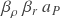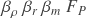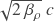# Formulas

The New Foundation Model reveals elementary forms of equations describing the physical attributes of elementary particles and systems. It also shows the relationships between these formulas and historical constants & equations.

Natural equations follow a pattern in which maximum potentials of the fundamental unit dimensions are diluted by proportionality operators resulting in the right quantity of a physical property.

## Mechanical formulas

The particle mechanics template illustrates which operators to apply to the maximum energy potential in each unit dimension.

Quantities of wavelength, mass, momentum, and energy are determined by applying the correct operators to the maximum potentials in these unit dimensions.

Kinetic energy unit dimensions are organized according to the 2-part energy mechanism–wavelength and velocity. The primary form of the formulas uses the wavelength operator for Part I and the velocity operator for Part II. Additional formulas can be constructed using the rest mass and momentum operators for Part I.

### Wavelength formula

The elementary particle wavelength formula applies the wavelength operator to the Planck length in Part I of the 2-part energy mechanism.

The simple formula for calculating the wavelength of an elementary particle with or without rest mass is

The formula leverages the inverse relationship between wavelength and inertial mass to find the wavelength given a quantity of inertial mass. There are many equivalent forms of the wavelength formula.

### Mass formula

The elementary formula for inertial mass applies the wavelength operator to the Planck mass in Part I of the 2-part energy mechanism.

Given a particle wavelength, inertial mass can be determined for particles with or without rest mass by multiplying the wavelength operator and the Planck mass.

While rest mass and inertial mass are different physical phenomena, rest mass can also be calculated using the wavelength operator in the special case of a particle’s Compton wavelength. For a particle with rest mass, the Compton wavelength is found at the limit of the particle’s velocity potential.

There are many equivalent forms of the mass formula.

### Momentum formula

Momentum formulas in traditional units of LMT-1 apply the wavelength operator to the Planck momentum potential in all three unit dimensions..

Given the wavelength operator in any form, momentum for particles with and without rest mass can be determined by multiplying the wavelength operator by the Planck momentum.

There are many equivalent forms of the momentum formula.

### Energy formula

The kinetic energy of particles with and without rest mass is determined using the spin, wavelength, and velocity operators.

Applying the three operators to the unit dimensions shown in the template produces the correct kinetic energy of all particle types.

### With rest mass

There are many equivalent forms of the energy formula.

## Gravitational Formulas

The New Foundation Model follows the same pattern for quantifying gravitational field potentials. The following formulas apply dimensionless proportionality operators to the maximum potentials in the unit dimensions of a given physical property or dynamic.### Gravitational acceleration### Gravitational energy### Gravitational force### Escape velocity### Orbital velocity## Electromagnetic formulas

Quantifying the maximum charge potential in natural units of time produces a formula quantifying the electrostatic force between electric charges.